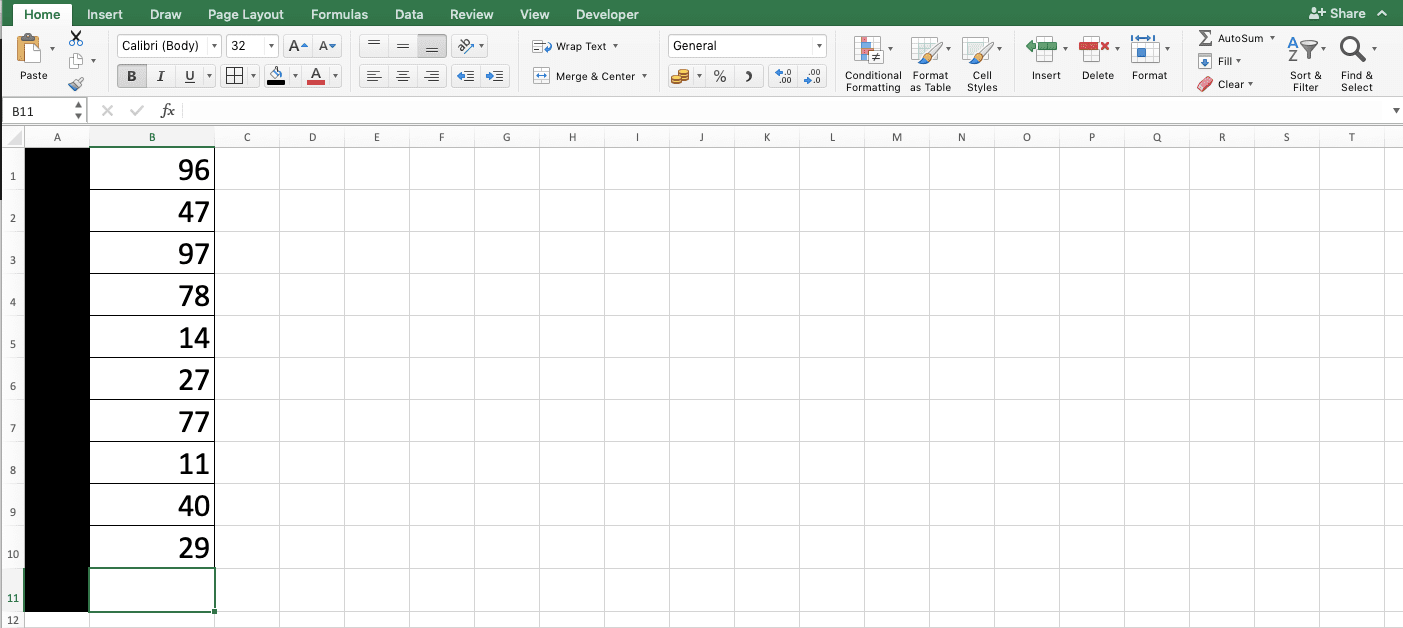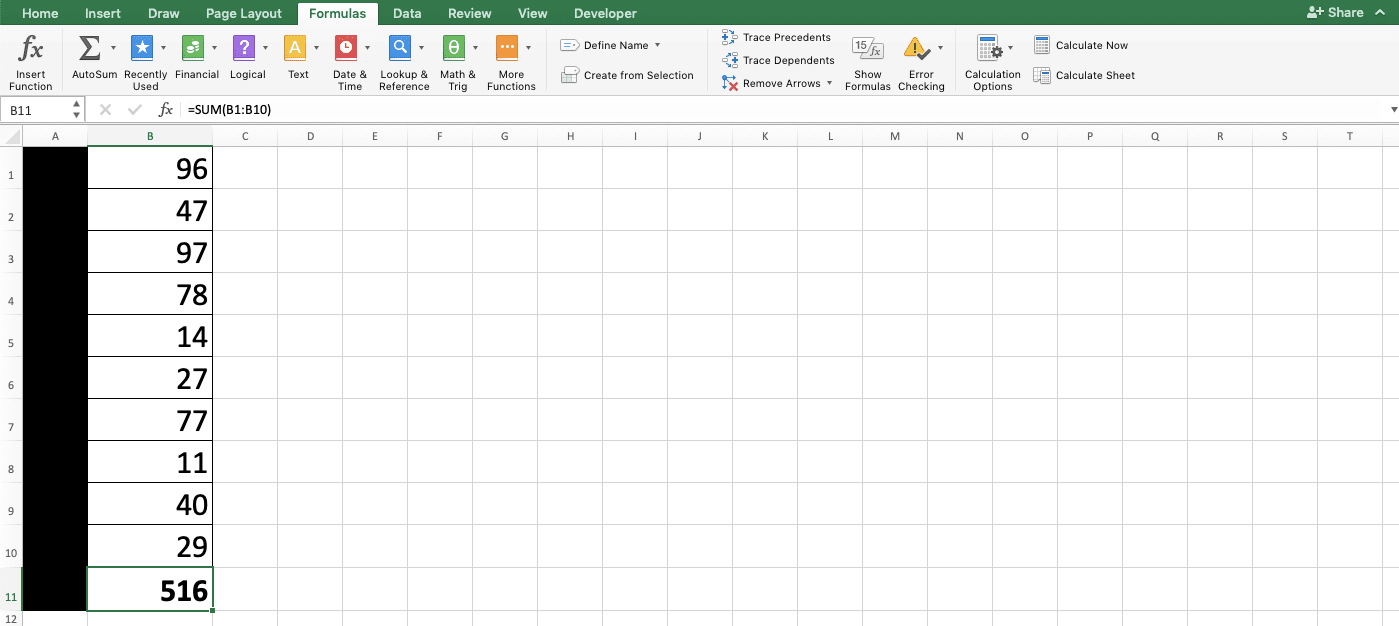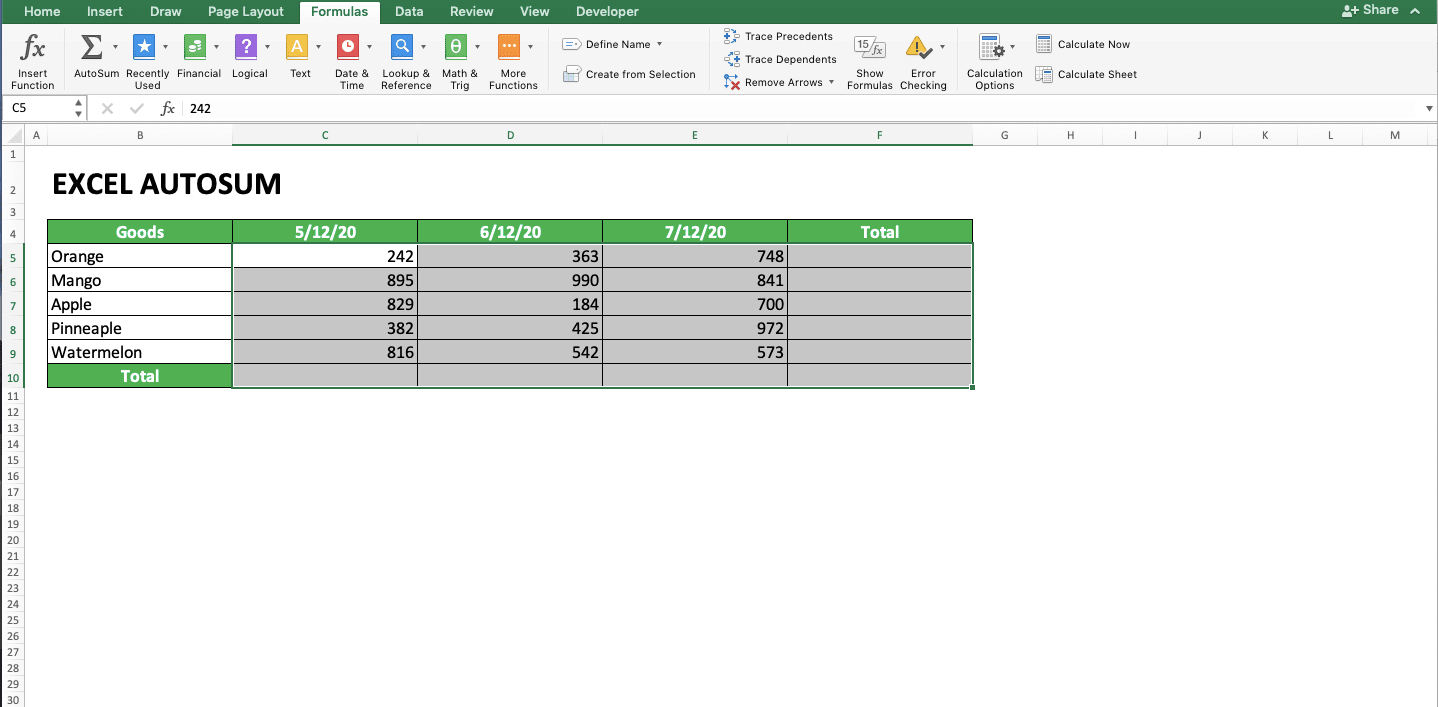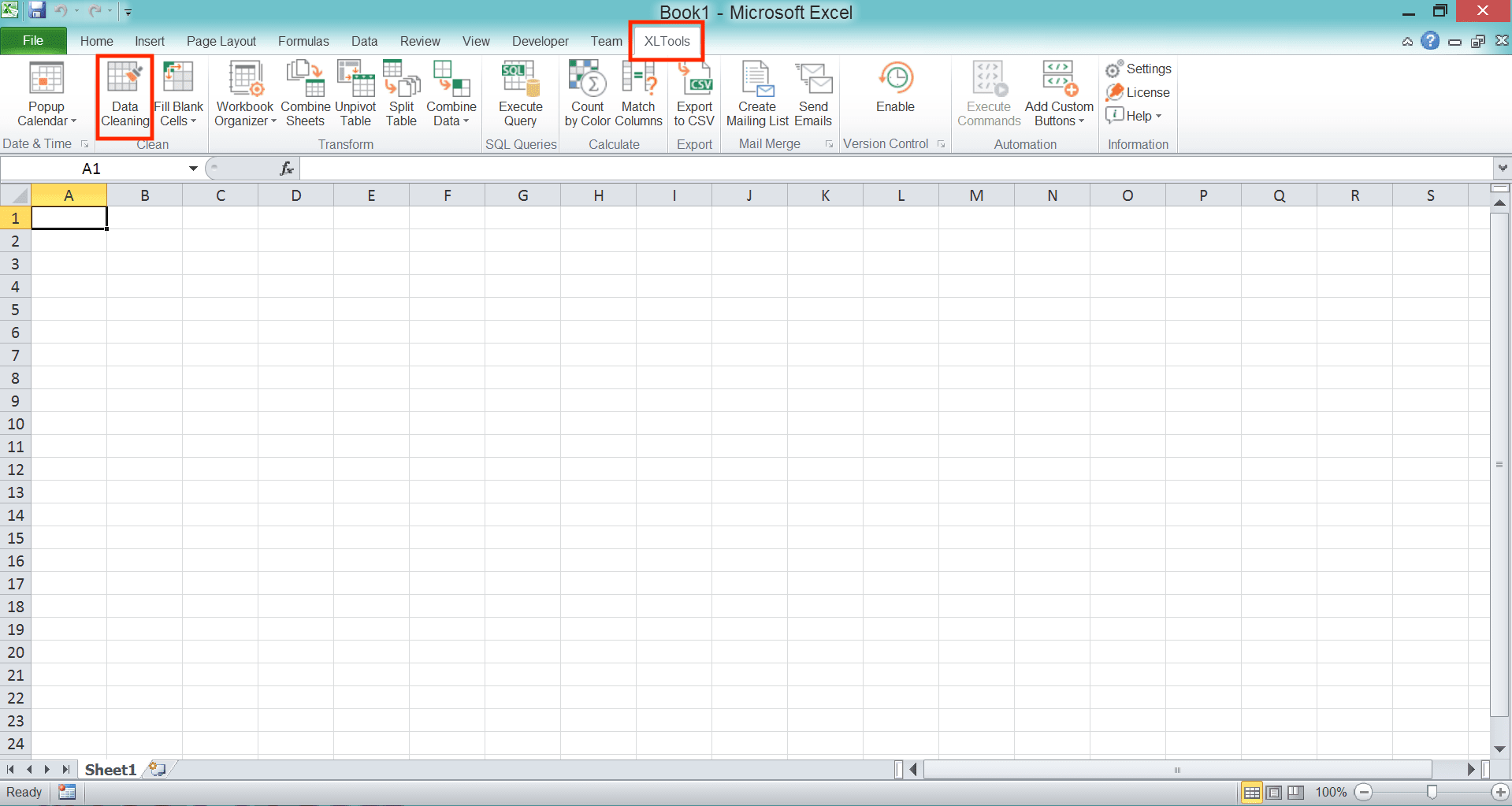How to Use AutoSum in Excel and Its Usability - Compute Expert

# How to Use AutoSum in Excel and Its Usability

Home >> Excel Tutorials from Compute Expert >> Excel Tips and Trick >> How to Use AutoSum in Excel and Its Usability

In this tutorial, we will discuss completely the AutoSum feature in excel. AutoSum is a feature that can be utilized if we want to sum numbers in sequence in a row/column.

Disclaimer: This post may contain affiliate links from which we earn commission from qualifying purchases/actions at no additional cost for you. Learn more

## AutoSum Usefulness

AutoSum can be used to help sum one series of numbers in a column/row fast. This feature will produce a SUM formula one cell outside your numbers series, with the input of all the series’ numbers.

## How to Use AutoSum

About its usage, briefly, you just need to put your cell cursor at the end of your numbers series. Then, choose the AutoSum menu. The menu itself can be accessed in the Home or Formula tab in your excel. The location of the AutoSum feature in each tab can be seen clearer below (in the red border-box, not the one around the tab, in each screenshot).## The Example of Its Usage and Result

The following will give and explain the examples of AutoSum usage and result in excel.In the example, you can see the AutoSum form after its activation to sum numbers in the sales quantity column. As seen in the formula bar, AutoSum produces the SUM formula to sum the numbers sequence in the sales quantity column.

The SUM formula which it produces is a normal SUM formula which you can change the inputs if needed. To produce this SUM formula, you just need to put your cell cursor at the end of your numbers sequence. Then, activate the AutoSum feature.

## Detailed Steps to Use AutoSum

The following will explain the steps to use AutoSum to make your understanding clearer about how to use this feature.

1. Place your cell cursor at the end of the sequence which contains all the numbers you want to sum2. Click the Home or Formulas Tab and click the AutoSum menu3. Press Enter
4. Done!Easy, isn’t it? With AutoSum, you can sum numbers in sequence in excel in less than one second!

## The AutoSum Shortcut

If you don’t like navigating to the Home or Formulas tab to activate the AutoSum, you can use its shortcut instead. Press the Alt and = buttons (Command, Shift, and T on Mac) simultaneously will activate the AutoSum feature for you.

## Other AutoSum Functions

Besides sum, you can also calculate the average, count numbers, and also find the largest and smallest value using AutoSum. Just put your cell cursor at the end of the numbers sequence and click the AutoSum menu dropdown. Choose:
• AVERAGE for the average calculation
• COUNT for the numbers count
• MAX for the largest value from your numbers sequence
• MIN for the smallest value from your numbers sequence

For a clearer understanding of how to access and use these four formulas using AutoSum, look at the screenshots below.Besides that, if you use a data filter, then you can use AutoSum to sum only the numbers shown by the filter. The sum on those numbers will happen automatically when you use it. If you use AutoSum at the end of the filtered numbers, then AutoSum will automatically produce SUBTOTAL (not SUM) to sum the numbers.## Sum More Than One Rows/Columns at Once With Different Results per Column/Row Using AutoSum

If you want to AutoSum many columns with per column result, you must highlight all cells after those numbers sequences. Then, just activate the AutoSum feature. The result will be the sum of the numbers sequence in each column for you!

To make the explanation clearer, take a look at the two screenshots below.The way is similar if you want to sum numbers sequences in some rows with per row result. Highlight the cells just outside the rows with the numbers. Then, activate the AutoSum feature.Here is another tip. If you want to sum your numbers per row and per column at once, highlight all the numbers. Highlight the cells just outside the rows and columns too. Then, activate the AutoSum feature.## Sum a Particular Cell Range from Your Number Sequence Using AutoSum

If you want to sum only parts of your numbers sequence using AutoSum, then highlight these parts first. Next, activate the AutoSum feature. Your sum result will be in one cell just outside the numbers sequence.## Things that Can Make AutoSum Doesn’t Produce the Correct Result

AutoSum won’t include numbers in its sum if the numbers:
• Have a text data type
• Are the results of other SUM formulas
• Above (column sequence)/on the left (row sequence) or in between the numbers with one of the two characteristics mentioned before

Because of that, if you use AutoSum and don’t get the results you expect, check your numbers. Probably, they have the characteristics mentioned above. If they are, then you must change them with normal numbers before using AutoSum so you can get the right result.

To convert text numbers into normal numbers in a cell range, you can use the Text to Columns feature in excel. Or if you have the XLTools Add-Ins, then use the Data Cleaning feature in the XLTools tab.

The way to convert is by highlighting the cell range containing your text numbers and use one of those features. You can see the Text to Columns and XLTools Data Cleaning locations below.

Text to Columns:Data Cleaning of the XLTools:## Exercise

After learning how to use the AutoSum feature in excel, now is the time to do an exercise. This is so you can sharpen your understanding of the use of the AutoSum feature!

### Question

Use the AutoSum to answer all the questions below! Give your answers in the gray-colored cells which fit the question numbers!
1. What is the sum of all numbers in column no. 1?
2. What is the average of all numbers in row no. 2?
3. What are the sum of all numbers in each row and column no. 3?

The AutoSum feature cannot be used to sum all numbers in more than one row or column.

Related tutorials you should learn:

Get updated excel info from Compute Expert by registering your email. It's free!